# Matrices

In Mathematics, matrices are arrays of numbers arranged in rows and columns.

### Row Matrix: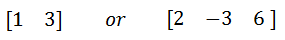### Coloumn Matrix:### Special Matrix:### Null Matrix (0):

Null Matrix is that matrix, that only contains number 0 in it.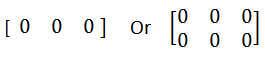### Diagonal Matrix:

Also known as square matrix, in which all element zero except the diagonal upper left to lower left.### Identity (or unit) Matrix (I):

The elements in the diagonal are one’s only.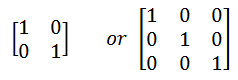### Writing the order of Matrices:

Order = Number of Rows *  Number of Columns

Example: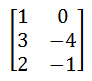Order = 3*2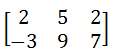Order = 2 * 3

### Addition and Subtraction of Matrices:

Matrices of the same order are added (or subtracted) by adding (or subtracting)  the corresponding elements in each matrix.Adding A + B:                     Subtracting A – B: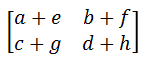Rules:

A+B = B+A : A and B can change position when adding.

(A+B) + C = A + (B+C): order of operation bracket first.

A – B ≠ B –A:  A and B should not change positions when subtracting.

Example:### Scaler Multiplication of a Matrix by a real number: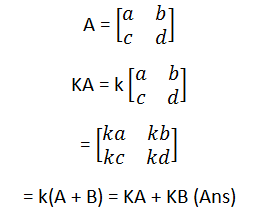Example:### Equal Matrix:

If two matrices A and B are of the same order and their corresponding elements are equal, then A = B.

Example: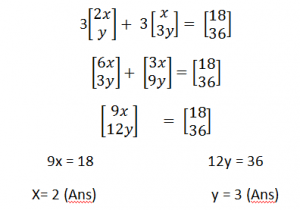### Multiplication of Two or More Matrices:

Matrices can only be multiplied only if they are compatible. They are compatible when the number of rows of the second matrix is the same as the number of coloumns of the first matrix.

Rules:

AB ≠ BA : A and B should not change positions

(AB)C = A(BC): If 3 or more matrices you can choose whichever 2 to    multiply first.

Example:### Inverse Of Matrix: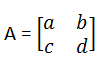Determinant A  = ad – bc

Remember:

• If Determinant = 0 then the matrix has no inverse.
• Multipying by the inverse of the inverse of a matrix gives the same result as dividing by the matrix.

E.g.

If AB = C

A-1AB = A-1C

B= A1C

Example of Inverse of Matrix:

• If Determinant = 0 then matrix has no inverse.
• Multipying by the inverse of the inverse of a matrix gives the same result as dividing by the matrix.

E.g.Example:

### 😃 Relax your Mind From Studying and WATCH this Beautiful Painting! 👇1.2.